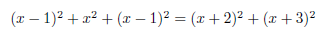### Article Index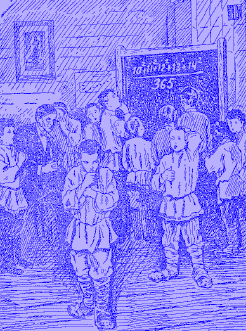Let us say somebody ask you to solve the next equation without using any technical aid.

Can you do this?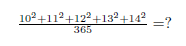O.k. it is not so easy. But when you know the special and interesting relation between those numbers:it is simple. The left components of the equation are: 100 + 121 + 144 = 365; In other words: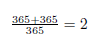O.k. let us figure out with the aid of little algebra if we can find more of such sequences: The first number we are looking for is “x”: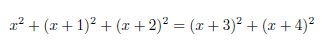or simple: